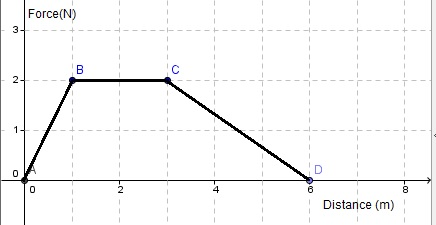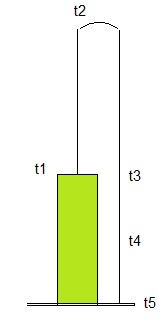# Solutions to Free SAT II Physics Practice Questions with Solutions Energy, Work and Power

 Solutions to Sat Physics subject questions on on energy, work and power with detailed explanations. How much work is done in pulling a box, on the ground, for 20 meters with a force of 20 Newtons making an angle of 10� with the ground? A) 400 J B) 4000 J C) 400 tan(10�) J D) 400 cos(10�) J E) 400 sin(10�) J Solution - Explanations work = force * distance cos (angle between force and direction of motion) = 20(N) 20 (m) cos (10�) = 400 cos (10�) A ball is thrown vertically upward with a velocity of 10 m/s. Calculate the maximum height reached by the ball before it returns to the ground. (g = 10 m/s2) A) 10 m B) 5 m C) 100 m D) 1 m E) 50 m Solution - Explanations Let v ( =10 m/s)be the initial velocity, m the mass of the ball, h the maximum height. When the ball has reached the maximum height, the velocity becomes zero and therefore all the Kinetic energy has become potential energy. PE = KE m g h = (1/2) m v 2 (g = 10 m/s 2) divide both sides by m and solve for h g = (1/2)(1/g) v 2 = (/20) 100 = 5 m When a car's speed changes from 30 m/s to 15 m/s, its kinetic energy A) is multiplied by 2 B) is divided by 2 C) is divided by 4 D) is multiplied by 4 E) does not change Solution - Explanations Note that from 30 to 15 m/s, the velocity is halved. Kinetic energy = (1/2) m v 2 (1) change v into v / 2 in the formula Kinetic energy = (1/2) m (v / 2) 2 = (1 / 2)(1 / 4) m v 2 Kinetic energy is divided by 4 Calculate the work done in pulling a box of 50 Kg up a 20� frictionless incline plane of length 15 m at a constant speed. A) 7500 sin (20�) J B) 7500 J C) 7500 tan (20�) J D) 750 J E) 7500 cos (20�) J Solution - Explanations Below is shown a diagram including the box and the forces acting on it. First the weight W of the box is decomposed into two components: Fx is the component parallel to the surface of the incline and Fy is the component perpendicular to the same surface. In order to pull the box, a force Fp opposite and equal in magnitude to Fx must be applied on the box (frictionless plane) in the direction of the surface of the incline plane.Fig1. - Box Pulled Up An Incline. Let L be the length of the incline plane. We first express the magnitude of Fx in terms of W and the angle as follows | Fx | = W sin (20�) magnitude of Fp | Fp | = | Fx | = W sin (20�) work done by Fp work = | Fp | L = L W sin (20�) = 15 (50 * 10) sin (20�) = 7500 sin (20�)Fig2. - Force Versus Distance Graph. Approximate the total work done by the force shown in the graph "force versus distance" on the right? A) 12 J B) 6 J C) 2 J D) 4 J E) 8 J Solution - Explanations The work is given by the area under the graph. It has the shape of a trapezoid. work = (1/2) (2) (6 + 2) = 8 j A ball is thrown upward at time t1 from the top of a building. At time t2 it reaches a maximum height and heads downward. At time t3 it is at height equal to the height of the building. At time t4, it is at a height equal to half the height of the building. It hits the ground at time t5. At what time is the potential energy of the ball maximum and it kinetic energy equal to zero? A) t1 B) t2 C) t3 D) t4 E) t5 Solution - Explanations A diagram of the trajectory is shown below. Before it is thrown, the ball already has potential energy proportional to the height of the building. When it is thrown upward, it will gain more potential energy (height increasing PE = m g h ) coming from the kinetic energy by throwing the ball. At t2, the ball stops and change direction: because it stops, its velocity is equal to zero hence its kinetic energy has been completely transformed into potential energy. At t = t2 the Kinetic energy is zero and the potential energy is maximum. Why maximum? because while falling the kinetic energy will increase and the potential energy start deceasing with the height to the ground.Fig1. - Trajectory. What is the power needed to lift a box of 200 Kg to a height of 20 meters, at a constant speed, in 20 seconds? A) 20,000 w B) 200 w C) 2000 w D) 200,000 w E) 2 w Solution - Explanations work needed to lift the box work = weight * height = 200 * 10 * 20 = 40,000 j power = work / time = 40,000 / 20 = 2000 watts A ball is thrown upward at time t1 from the top of a building. At time t2 it reaches a maximum height and heads downward. At time t3 it is at height equal to the height of the building. At time t4, it is at a height equal to half the height of the building. It hits the ground at time t5. In which interval of time is the potential energy of the ball increasing while its kinetic energy is decreasing? A) t1 , t2 B) t2 , t3 C) t3 , t4 D) t4 , t5 E) t3 , t5 Solution - Explanations Refer to the diagram in question 7. Between t1 and t2, the ball gain height and therefore its potential energy increase. This increase comes from the kinetic energy of the ball that is decreasing and then becomes zero at t2. The mass m and velocity v of each of 5 moving objects are given below. Which one has the highest kinetic energy? A) m = 10 Kg , v = 100 m/s B) m = 100 Kg , v = 10 m/s C) m = 1,000 Kg , v = 1 m/s D) m = 10,000 Kg , v = 0.1 m/s E) m = 100,000 Kg , v = 0.01 m/s Solution - Explanations Use the formula KE = (1 / 2) m v 2 and show that A) has the A forklift lifted a box of mass M at a constant speed to a height of 10 meters using a power of 1000 Watts in 20 seconds. Find mass M. A) 1 Kg B) 2000 Kg C) 20 Kg D) 200 Kg E) 100 Kg Solution - Explanations The work to be done by the forklift gives the box potential energy (gaining height). Hence the work W1 to be done by the forklift is equal to the total potential energy of the box once lifted. W1 = M g h = M (10)(10) = 100 M the power and time needed to lift the box are is also known, hence the work actually done by the forklift W2 = power * time = 1000 * 20 W1 and W2 are equal assuming no loss of energy (all energy developed by the forklift was efficiently used to lift the box) hence 100 M = 20,000 M = 200 Kg Answers to the Above questions D B C A E B C A A D